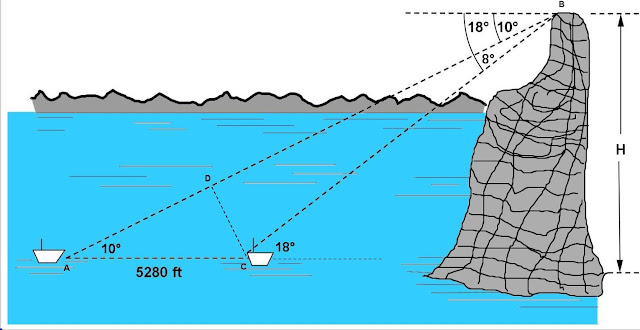## Thursday, February 28, 2013

### Word Problem - Partition, Distribution

Category: Algebra

"Published in Newark, California, USA"

Alberto would like to distribute a bagful of marbles equally among his friends. He figures out that if he were to give them 7 marbles each, he will need 4 marbles more but if he were to give them 6, he will have 3 marbles left. How many marbles does Alberto have?

Solution:

For this type of word problem, we will use the principles of linear equation where the partition or distribution of items is involved. Let's analyze the given word problem as follows

Let x be the number of marbles
y be the number of friends to distribute the marbles

If the statement says "if he were to give them 7 marbles each, he will need 4 marbles more", then the working equation will be

or

If the statement says "if he were to give them 6, he will have 3 marbles left", then the working equation will be

or

Equate x = x, we have

Therefore,

Substitute the value of y to either one of the two equations, we have

Therefore, Alberto has 294 marbles that he distributed to his 46 friends

## Wednesday, February 27, 2013

### Escape Velocity - Solar System, Planet

Category: Differential Equations, Physics

"Published in Newark, California, USA"

The radius of the moon is roughly 1080 miles. The acceleration of gravity at the surface of the moon is about 0.165g, where g is the acceleration of gravity at the surface of the earth. Determine the velocity of escape for the moon. (Hint: g = 32.16 ft/sec2 and 1 mile = 5280 ft)

Solution:

Consider the problem of determining the velocity of a particle projected in a radial direction outward from the earth and acted upon by only one force, the gravitational attraction of the earth.

We shall assume an initial velocity in a radial direction so that the motion of the particle takes place entirely on a line through the center of the earth.

According to the Newtonian Law of Gravitation, the acceleration of the particle will be inversely proportional to the square of the distance from the particle to the center of the earth. Let r be that variable distance, and let R be the radius of the earth. If t represents time, v is the velocity of the particle, a its acceleration, and k is the constant of proportionality in the Newtonian Law, then

The acceleration is negative because the velocity is decreasing. Hence the constant k is negative. When r = R, then a = - g, the acceleration of gravity at the surface of the earth. Thus

or

from which

We wish to express the acceleration in terms of the velocity and the distance. We have a = dv/dt and v = dr/dt. Hence

so the differential equation for the velocity is now seen to be

The method of separation of variables applies to equation above and leads at once to the set of solutions

or

Suppose that the particle leaves the earth's surface with the velocity v0. Then v = v0 when r = R, from which the constant C is easily determined to be

Thus, a particle projected in a radial direction outward from the earth's surface with an initial velocity v0 will travel with a velocity v given by the equation

If v becomes zero, the particle would stop, the velocity would change from positive to negative, and the particle would return to the earth.

If r becomes very large, then the first term will be very small and even equal to zero.

To avoid that v becomes zero, then the next two terms in the equation above must be

If

then we can solve for the value of v0 as follows

or

The above equation is called the velocity of escape. This is applicable at any planets and moons in a solar system including earth.

Now, let's go back to the given problem that if the radius of a moon is 1080 miles and the acceleration of gravity is 0.165g, then the velocity of escape at the moon will be equal to

or

## Tuesday, February 26, 2013

### Angle - Depression Problem

Category: Trigonometry

"Published in Newark, California, USA"

A surveyor on a mountain peak observes below him two vessels lying at anchor 1 mile apart and in the same vertical plane with his position. He finds the angles of depression of the ships to be 18° and 10° respectively. How high does the peak rise above the water? (1 mile = 5280 feet)Photo by Math Principles in Everyday Life

Solution:

This is also a great application of angle of depression especially for the surveyors and Civil Engineers. Let's analyze and label further the above figure as followsPhoto by Math Principles in Everyday Life

but

The above equation becomes

but

Therefore, the height of a mountain peak from the water is